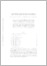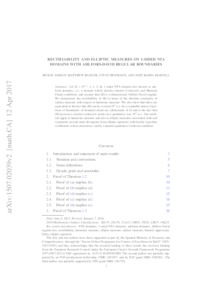# Rectifiability and elliptic measures on 1-sided NTA domains with Ahlfors-David regular boundaries

Akman, Murat and Badger, Matthew and Hofmann, Steve and Martell, José María (2019) 'Rectifiability and elliptic measures on 1-sided NTA domains with Ahlfors-David regular boundaries.' Transactions of the American Mathematical Society, 369 (8). pp. 5711-5745. ISSN 0002-9947Preview
Text
1507.02039v2.pdf - Accepted Version

Let $\Omega\subset\mathbb{R}^{n+1}$, $n \geq 2$, be 1-sided NTA domain (aka uniform domain), i.e.~a domain which satisfies interior Corkscrew and Harnack Chain conditions, and assume that $\partial\Omega$ is n-dimensional Ahlfors-David regular. We characterize the rectifiability of $\partial\Omega$ in terms of the absolute continuity of surface measure with respect to harmonic measure. We also show that these are equivalent to the fact that $\partial\Omega$ can be covered $\mathcal{H}^{n}$-a.e. by a countable union of portions of boundaries of bounded chord-arc subdomains of $\Omega$ and to the fact that $\partial\Omega$ possesses exterior corkscrew points in a qualitative way $\mathcal{H}^{n}$-a.e. Our methods apply to harmonic measure and also to elliptic measures associated with real symmetric second order divergence form elliptic operators with locally Lipschitz coefficients whose derivatives satisfy a natural qualitative Carleson condition.View Item